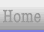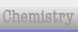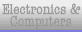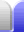# Multiplying and Dividing Fractions

### Multiplying Fractions

To multiply two fractions together multiply the numerators together to find the numerator of the answer, and multiply the denominators together to find the denominator of the answer.

12 x 32 = 34

Because multiplication is commutative we can multiply the fractions in any order.

 1 x 7 x 2 = 1 x 2 x 72 2 5 2 5 2

We can also multiply the numerators in any order, and the denominators in any order, and because multiplication is also associative and distributive, we can break out any fraction we like.

 1 x 7 x 2 = 1 x 7 x 2 = 1 x 7 x 2 = 1 x 7 x 22 2 5 2 x 2 x 5 2 x 5 x 2 2 x 5 2

Notice that last step? We have a fraction where the numerator is the same as the denominator. Any number (except zero) divided by itself is 1. Any number multiplied by 1 remains unchanged. So we have

 1 x 7 = 72 x 5 10

This saves us from having to work out the whole thing then factorise the answer to see if we can simplify it. We need to find common factors – factors that both the numerator and denominator have.

 1 x 7 x 2 = 1 x 7 x 2 = 14 = 2 x 7 = 72 2 5 2 x 2 x 5 20 2 10 10

### Reciprocals & Dividing Fractions

The reciprocal of a number is 1 divided by the number, so the reciprocal of 2 is 12. To find the reciprocal of a fraction, swap the numerator and the denominator.

Any number multiplied by its reciprocal equals 1.

79 x 179 = 7979 = 1

Reciprocals of fractions are very useful because dividing by a number is the same as multiplying by its reciprocal.

12 ÷ 23 = 12 x 32 = 34

Let's have another look at that example of a compound fraction.

 1⁄17 = 1 ÷ 3 = 1 x 5 = 53⁄5 17 5 17 3 51Home Astronomy Chemistry Electronics & Computers Mathematics Physics Field Trips Turn on javascript for email link